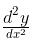# Test: Difference Of Parametric Functions

## 10 Questions MCQ Test Mathematics (Maths) Class 12 | Test: Difference Of Parametric Functions

Description
This mock test of Test: Difference Of Parametric Functions for JEE helps you for every JEE entrance exam. This contains 10 Multiple Choice Questions for JEE Test: Difference Of Parametric Functions (mcq) to study with solutions a complete question bank. The solved questions answers in this Test: Difference Of Parametric Functions quiz give you a good mix of easy questions and tough questions. JEE students definitely take this Test: Difference Of Parametric Functions exercise for a better result in the exam. You can find other Test: Difference Of Parametric Functions extra questions, long questions & short questions for JEE on EduRev as well by searching above.
QUESTION: 1

### Differentiate sin2(θ2 + 1) with respect to θ2

Solution:

y = sin22+1)
v = θ2
dy/d(v) = dydθ/dvdθ
dy/dthη = sin2(V+1)
= 2sin(V+1)⋅cos(V+1)dv/dθ
= 2sin(θ2+1)cos(θ2+1)
= sin2(θ2+1).

QUESTION: 2

Solution:
QUESTION: 3

### Find dy/dx if x= a cos θ, y = b sin θ

Solution:
QUESTION: 4

Difference equation in discrete systems is similar to the _____________ in continuous systems.

Solution:

Difference equation are the equations used in discrete time systems and difference equations are similar to the differential equation in continuous systems.

QUESTION: 5

X = at² and y = 2at are parametric equations of

Solution:

Together the equations x = at2 and y = 2at (where t is the parameter) are called the parametric equations of the parabola y2 = 4ax.

QUESTION: 6

If x = 4(t + sin t), y = 4(1-cos t), Evaluate dy/dx at t= π/2

Solution:

x = a(t+sin t)
⟹dx/dt = a(1+cos t)
And y = a(1−cos t)
⟹dy/dt = a[0−(−sin t)]
=a sin t
Therefore, dy/dx = a sin t/(a(1+cos t))
= 2sin t/2 cos t/2)/(2cos^2 t/2)
=tan(t/2)
At π/2

tan(π/4) = 1

QUESTION: 7

Find dy/dx if x = cos3 θ, y = sin3 θ

Solution:

x = cos3θ
⇒ dx/dθ = a(3cos2θ)(−sinθ)
= −3sinθcos2θ
y = sin3θ
⇒dy/dθ = a(3sin2θ)(cosθ)
= 3sin2θcosθ
∴dy/dx = (dy/dθ)(dx/dθ)
= (3sin2θcosθ)/(−3asinθcos2θ)
= −sinθ/cosθ
= −tanθ

QUESTION: 8

Find; x = 20 (cos t + sin t) and y = 20 ( sin t - t cos t)

Solution:

x = 20(cost + tsint)
differentiate x with respect to t,
dx/dt = 20{d(cost)/dt + d(tsint)/dt]
= 20[-sint + {t. d(sint)/dt + sint.dt/dt}]
= 20[ -sint + tcost + sint]
= -20t.cost
hence, dx/dt = -20t.cost -----(1)

y = 20(sint - tcost)
differentiate y with respect to t,
dy/dt = 20[d(sint)/dt - d(tcost)/dt ]
= 20[cost - {t.d(cost)/dt + cost.dt/dt}]
= 20[cost +tsint -cost]
= 20t.sint
hence, dy/dt = 20t.sint -------(2)

dividing equations (2) by (1),
dy/dx = 20t.sint/20t.cost
dy/dx = tant
now again differentiate with respect to x
d²y/dx² = sec²t. dt/dx ------(3)
now from equation (1),
dx/dt = 20t.cost
so, dt/dx =1/at.cost put it in equation (3),
e.g., d²y/dx² = sec²t. 1/20t.cost
d²y/dx² = sec³t/20t

QUESTION: 9

If f (x) = [x sin p x] { where [x] denotes greatest integer function}, then f (x) is

Solution:

If −1≤x≤1, then 0≤xsinπx≤1/2
​∴ f(x)=[xsinπx]=0, for −1≤x≤1
If 1<x<1+h, where h is a small positive real number, then
π<πx<π+πh
⇒−1<sinπx<0
⇒−1<xsinπx<0
∴ f(x)=[xsinπx]=−1 in the right neighbourhood of x=1.
Thus, f(x) is constant and equal to zero in [−1,1] and so f(x) is differentiable and hence continuous on (−1,1).

QUESTION: 10

Differentiate sin x3 with respect to x3

Solution:

Differentiation of sinx3 with respect to x3 is
d(sin(x3)/dx = cosx3